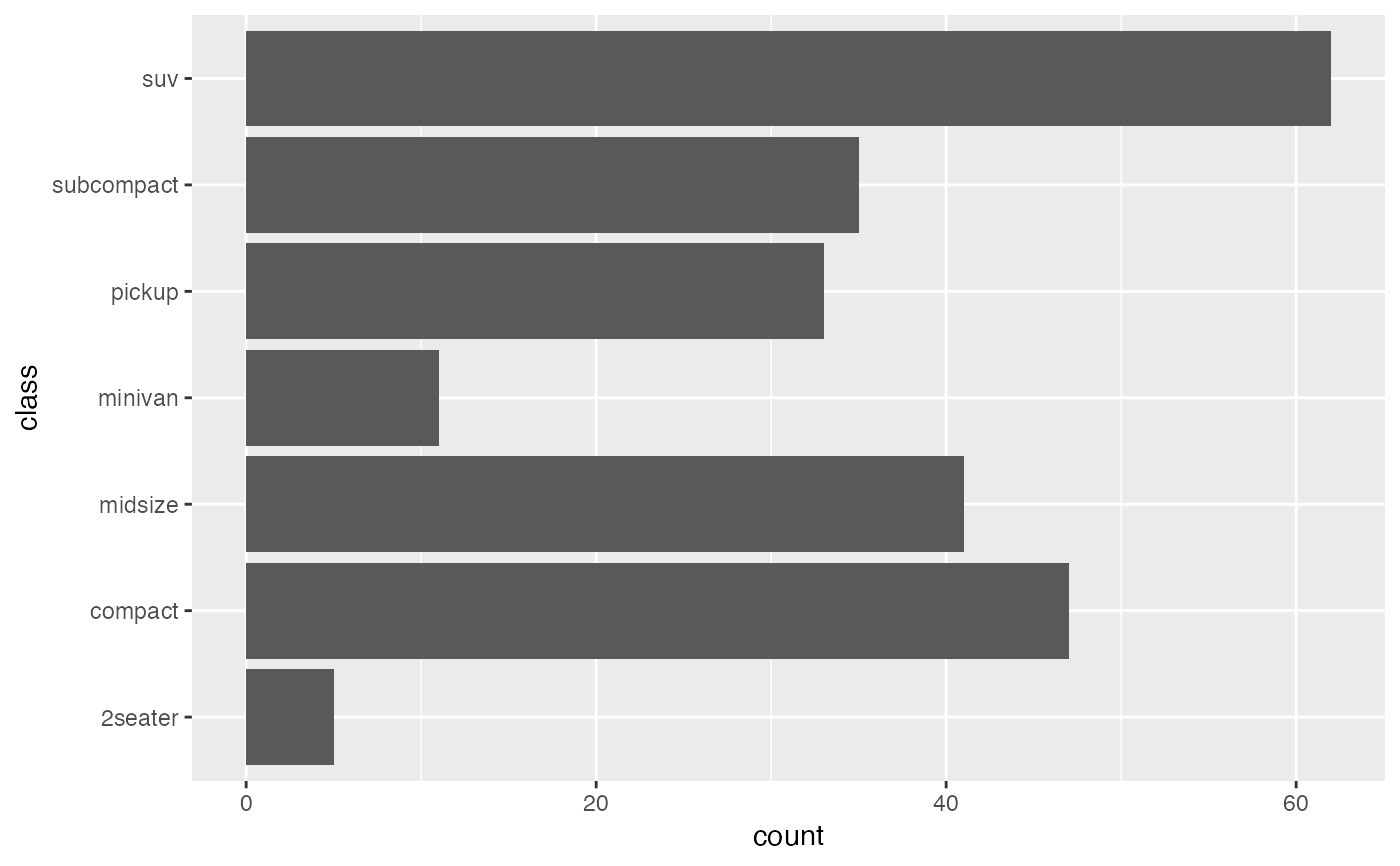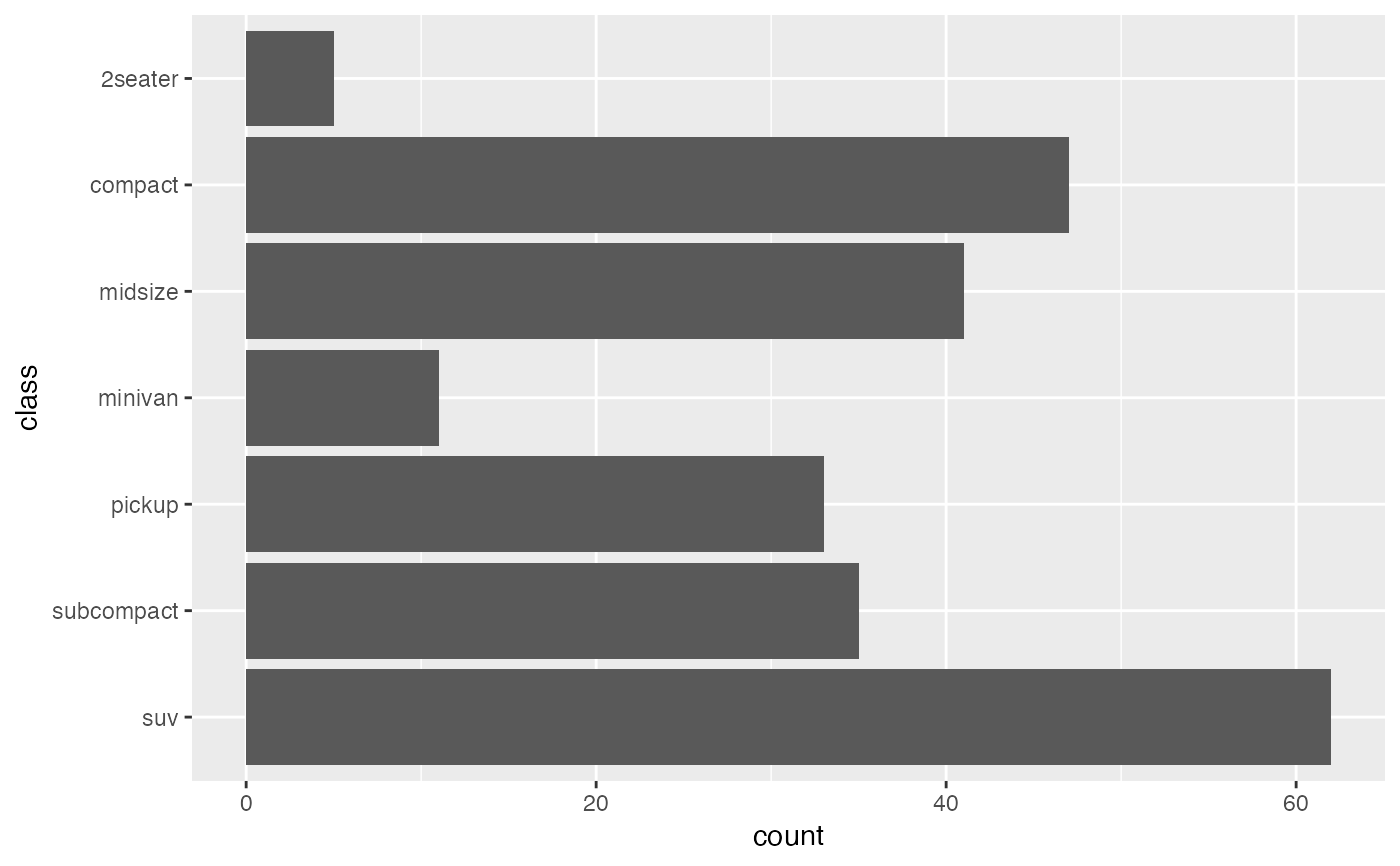Intelligently flip a plot with discrete data on the X axis.

## Usage

acid_discrete_coord_flip()

## Value

list of ggproto objects.

## Details

This function puts the samples that were near the left origin on the X at the top on the Y axis, making them more human readable.

## Note

Updated 2023-08-11.

• coord_flip().

## Examples

library(ggplot2)
g <- ggplot(data = mpg, aes(x = class)) +
geom_bar()
## Notice the difference in Y axis sample order.
g + coord_flip()g + acid_discrete_coord_flip()